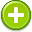#percentage 中文解釋 wordnet sense Collocation Usage
Noun
/pərˈsentij/,Font size:percentages, plural;
1. A rate, number, or amount in each hundred
• - the percentage of cesareans at the hospital was three percent higher than the national average
• - a large percentage increase in the population over 85
2. An amount, such as an allowance or commission, that is a proportion of a larger sum of money
• - I hope to be on a percentage
3. Any proportion or share in relation to a whole
• - only a small percentage of black Americans have Caribbean roots
• - you explain to me the percentage in looking like a hoodlum

1. a proportion in relation to a whole (which is usually the amount per hundred)
2. share: assets belonging to or due to or contributed by an individual person or group; "he wanted his share in cash"
3. In mathematics, a percentage is a way of expressing a number as a fraction of 100 (per cent meaning "per hundred" in Latin). It is often denoted using the percent sign, "%", or the abbreviation "pct". For example, 45% (read as "forty-five percent") is equal to 45/100, or 0.45.
4. The amount, number or rate of something, regarded as part of a total of 100; a part of a whole; A share of the profits; Benefit or advantage
5. (Percentages) (Playing the) - A player is "playing the percentages" when he chooses shots that he has a reasonably good chance of successfully completing - provided he has the option to choose.
6. (Percentages) The user enters any two of the values---whole, part, and percent---and clicks on Compute. Although the computer does the work, the applet nicely models percent problems.
7. Percentages are simple sums divided by totals or normalised on a population (e.g., percentage of installations that have submitted safety reports, percentage staff whose performance during emergency response exercise was “good” or “very good”); and
8. a way of measuring. The number 100 (which stands for the whole amount) is usually divided into 100 smaller, but equal, parts, each called a percent. So a percentage usually refers to a certain number of parts within the whole. Therefore, 6% is 6 units out of 100% (the whole). ...
9. The amount of coverage in a halftone dot is called Percentage or Tint. A 10% dot is much smaller than an 80% dot. When screen printed, a dot grows in size. This is called dot gain and it can be as much as 30% when printing on an automatic press and 40% when using a manual. ...
10. The number of units with a certain characteristic divided by the total number of units in the sample and multiplied by 100. For example, the percentage that represents five out of 20 boys is 5 divided by 20 x 100, which is 25%.
11. Calculated based on the records displayed (not total records) for a particular report. When a report's count value changes, we recalculate percentages.
12. Older dictionaries suggested that percentage be used when a non-quantitative statement is being made: 'The percentage growth of the economy was encouraging.' But use percent when specifying a numerical value: 'The gross national product increased by 2 percent last year. ...
13. An amount, number, or rate stated as a proportion of a whole.
14. A part of a whole expressed in hundredths.
15. A fraction expressed in hundredths. If 50% of people are male, then 50 out of every 100 people are male. But beware of surveys with small sample sizes. If 2 people were surveyed, and 1 was male, that's still 50%. ...
16. A percentage measures a figure out of a hundred parts, and locates a number in relation to the highest possible score. In tests a percentage score measures the student’s score in relation to the highest possible score. For example, if a student gets 90 out of 100 on a test, they wouls score 90%.
17. Per the laws in the United Kingdom each fruit machine has got to pay out a certain amount of money and the minimum percentage is 70%. The goal of the machine is to meet this percentage over a large number of credits / spins. ...
18. The result of multiplying any ratio by 100.
19. Also known as PC (percent casino). The percent of all wagers the house can expect to win, over a period of time.  Can vary widely, but normally is about 30%.
20. most miners and employees work on prearranged percentages of the proceeds of mining
21. Generally, rate per hundred. Expressed by the mark '%'. See also 'Index'.
22. literally 'per hundred', percentages are an expression of a number as its proportion of 100 as in "47% of the population will vote Labour in the next election".
23. Amount of your bet HOUSE keeps by paying you at less that true odds when you win.
24. The hidden or direct charge made by the casino.
25. In South Australia, ladder percentage is usually calculated as "For" ÷ "For and Against" × "100". Elsewhere in Australia it is generally calculated as "For" ÷ "Against" × "100".